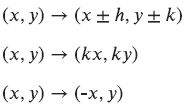# G.6.2.3 What Does it Do?

Below are some transformation rules (a-c). Apply each rule to quadrilateral on the graph above and graph the resulting image. You will need to scroll to the bottom of the input bar to start inputting your values (coordinate pairs). Once you enter the 4 coordinates, use the polygon tool to connect the coordinates you plot. The polygon tool will display confusing entries in the input bar (as you can see above), don't worry about them. a. (x, y)(2x, y) b. (x, y)(x, -y) c. (x, y)(y, -x) Then give a detailed description of the transformations; use one of these phrases in your detailed description: horizontal streatch, vertical stretch, reflection, rotation, or dilation.

In the previous activity, one rule was (x, y)(2x, 2y). In this activity, one rule was (x, y)(2x, y). Compare and contrast these rules.

Can we call (x, y)(2x, y) a dilation? Why or why not?”

Can we call (x, y)(2x, y) a translation? Why or why not?

The rule (x, y)(x, -y) is a reflection across the x-axis. Why does this rule produce a reflection?”

h and k represent numbers. What do you think each rule represents?